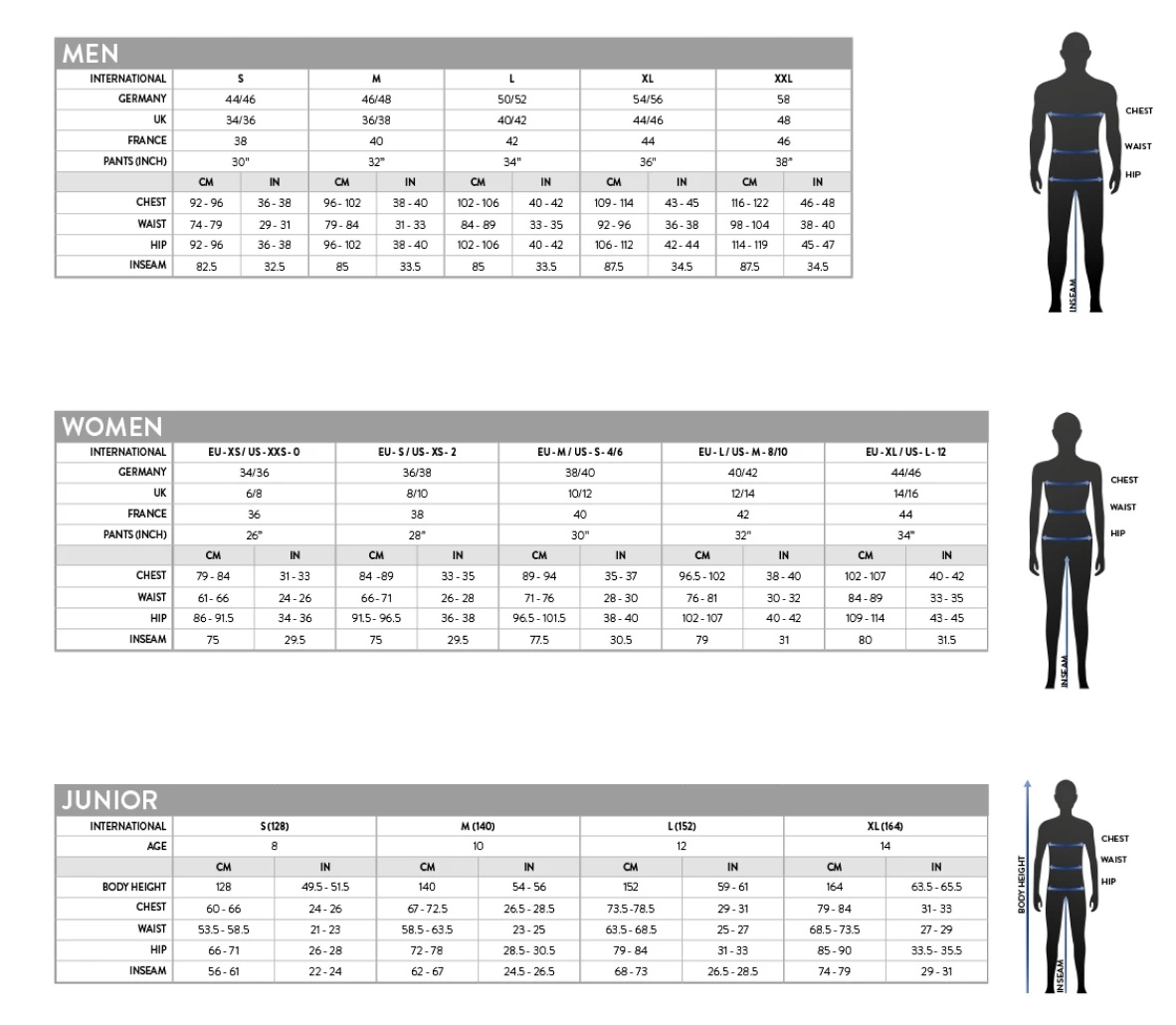Available on this page are a useful chart and calculator for anyone looking to determine their torsion bars equivalent wheel rate. Wheel rate is the spring rate of a theoretical spring attached directly above your wheel. Identical to spring rate, wheel rate is measured in lbs/in. To keep our measurements consistent, we make a few assumptions in our calculations.

• Torsion bar arm is infinitely rigid and does not flex. (In real world applications, arm flex slightly contributes to wheel rate.)
• We look at one inch of wheel travel while the arm is parallel to the ground. (As your arm pivots and the angle with the ground increases, the mathematical moment arm decreases, and wheel rate increases.)
• We do not account for tire data. (Tires have their own spring rate and will affect rate.)
• We do not account for flex in any of the suspension or chassis components that will contribute to wheel rate.

With these assumptions we are able to isolate a torsion bars effective wheel rate and make accurate comparisons. Below are the equations we use behind the scenes in our calculator and the one we used to generate our wheel rate chart

Kwheel = Wheel Rate [lb/in]

X = Wheel Travel [in]

J = Polar Moment of Inertia [in4]

G = Shear Modulus [lb/in2]

L = Torsion Bar Active Length [in]

d = Arm Length [in]

Do = Outside Diameter [in]

Di = Inside Diameter [in]

J=\frac{\pi(D_o^4-D_i^4)}{32}&s=3$ K_{wheel}=\frac{\tan^{-1}(\frac{X}{d})JG}{XLd}&s=3$